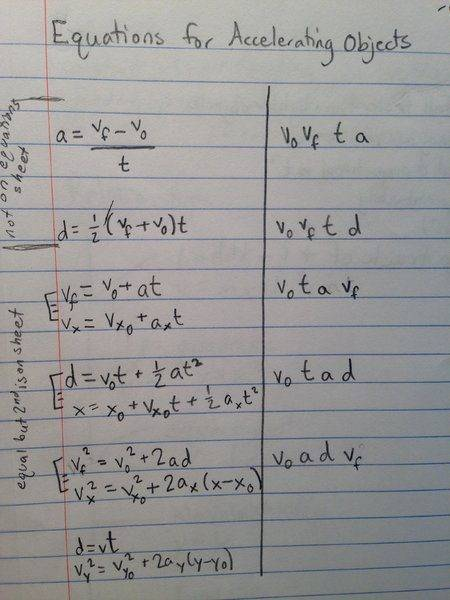# Velocity falling from window

Wanting to Learn

## Homework Statement

Roger sees water balloons fall past his window. He notices that each balloon strikes the sidewalk 0.83 s after passing his window. Roger's room is on the third floor, 15 m above the sidewalk.
Part A:
How fast are the balloons traveling when they pass Roger's window? (express to 3sig figs with units)
Part B:
Assuming the balloons are being released from rest, from what floor are they being released? Each floor of the dorm is 5.0 m high. (Express answer as integer. Thus the balloons are coming from the floor number _.)

d = .5(vf + v0)t

vf = 0m/s
v0 = ? m/s
t = 0.83s
d = 15m

## The Attempt at a Solution

I plugged in:
15 = .5(0 + v)*0.83
15 = 0.415v
v = 36.14...
I assume units are m/s

When I put 36.1 m/s as the answer (homework is online) it said my answer is incorrect. Where did I do wrong with it?
(I will attempt part B once I have the correct answer for part A.)

Thank you in advance for helping.

## Answers and Replies

TSny
Homework Helper
Gold Member
vf = 0m/s
Is this the final velocity of the balloon just before striking the ground?

Wanting to Learn
Is this the final velocity of the balloon just before striking the ground?
Maybe not? I would think that the water balloon keeps getting faster, at least until a certain speed, as it falls, and velocity is 0 when it has hit the ground. Perhaps I need to not use that and use a different equation? (In class we were given a list of equations for accelerating objects but I'm not always sure which one to use.)

Wanting to Learn
These are the equations we were given:The right column lists the variables used.

vela
Staff Emeritus
Science Advisor
Homework Helper
Education Advisor
Maybe not? I would think that the water balloon keeps getting faster, at least until a certain speed, as it falls, and velocity is 0 when it has hit the ground. Perhaps I need to not use that and use a different equation? (In class we were given a list of equations for accelerating objects but I'm not always sure which one to use.)
I think you can safely neglect air resistance, so the balloons will be speeding up the entire time.

As I like to ask my students, "Imagine you are the ground. Would you say the balloons were going 0 m/s when they hit you?"

•TSny
Wanting to Learn
Okay, so should I try using this equation?
d = v0t + .5at2
so
15 = 0.83v + .5*9.8*0.832
4.4436... = 0.83v
v = 3.6882...

EDIT: 3.69 m/s is not the correct answer, I'm still not sure what I've been doing wrong or if I need to use a different equation

Wanting to Learn
I've gotten the correct answer to Part A, turns out I had the correct equation though perhaps typed something incorrectly in the calculator. The answer to Part A is 14.0m/s. I will attempt part B later and post again if I get it or need help.

TSny
Homework Helper
Gold Member
I've gotten the correct answer to Part A, turns out I had the correct equation though perhaps typed something incorrectly in the calculator. The answer to Part A is 14.0m/s. I will attempt part B later and post again if I get it or need help.
OK. Your work for part A looks good.

Wanting to Learn
Thank you, I did manage to get Part B